# pygimli.meshtools¶

Mesh generation and modification.

Note

Although we discriminate here between grids (structured meshes) and meshes (unstructured), both objects are treated the same internally.

## Overview¶

Functions

 appendTetrahedronBoundary(mesh[, xbound, …]) Return new mesh surrounded by tetrahedron boundary box. appendTriangleBoundary(mesh[, xbound, …]) Add a triangle mesh boundary to a given mesh. cellDataToBoundaryData(mesh, data) TODO DOCUMENT_ME cellDataToNodeData(mesh, data[, style]) Convert cell data to node data. convert(mesh[, verbose]) Convert mesh from foreign formats. convertHDF5Mesh(h5Mesh[, group, indices, …]) Converts instance of a hdf5 mesh to a GIMLI::Mesh. convertMeshioMesh(mesh[, verbose]) Convert mesh from meshio object. createCircle([pos, radius, nSegments, …]) Create simple circle polygon. createCube([size, pos, rot, boundaryMarker]) Create cube PLC as geometrie definition. createCylinder([radius, height, nSegments, …]) Create PLC of a cylinder. createFacet(mesh[, boundaryMarker, verbose]) Create a coplanar PLC of a 2d mesh or poly createGrid([x, y, z]) Create grid style mesh. createGridPieShaped(x[, degree, h, marker]) Create a 2D pie shaped grid (segment from annulus or cirlce). createLine(start, end[, nSegments]) Create simple line polygon. createMesh(poly[, quality, area, smooth, …]) Create a mesh for a given PLC or point list. Generate simple one dimensional mesh with nodes at position in RVector pos. createMesh1DBlock(nLayers [[, nProperties]) Generate 1D block model of thicknesses and properties createMesh2D(x, y [[, markerType]) Generate simple two dimensional mesh with nodes at position in RVector x and y. createMesh3D(x, y, z [[, markerType]) Generate simple three dimensional mesh with nodes at position in RVector x and y. createMeshFromHull(mesh[, fixNodes]) Create a new 2D triangular mesh from the boundaries of mesh. createParaDomain2D(*args, **kwargs) API change here .. createParaMesh(*args, **kwargs) Create parameter mesh from list of sensor positions. createParaMesh2DGrid(sensors[, paraDX, …]) Create a grid-style mesh for an inversion parameter mesh. createParaMeshPLC(sensors[, paraDX, …]) Create a PLC mesh for an inversion parameter mesh. createPolygon(verts[, isClosed, addNodes, …]) Create a polygon from a list of vertices. createRectangle([start, end, pos, size]) Create rectangle polygon. createWorld(start, end[, marker, area, …]) Create simple rectangular world. exportFenicsHDF5Mesh(mesh, exportname) Exports Gimli mesh in HDF5 format suitable for Fenics. exportHDF5Mesh(mesh, exportname[, group, …]) Writes given GIMLI::Mesh in a hdf5 format file. exportPLC(poly, fname, **kwargs) General writer to save a piece-wise linear complex (PLC), i.e. exportSTL(mesh, fileName[, binary]) Write STL surface mesh and returns a GIMLI::Mesh. extrude(p2[, z, boundaryMarker]) Create 3D body by extruding a closed 2D poly into z direction extrudeMesh(mesh, a, **kwargs) Extrude mesh to a higher dimension. fillEmptyToCellArray(mesh, vals[, slope]) Prolongate empty cell values to complete cell attributes. interpolate(*args, **kwargs) Interpolation convinience function. interpolateAlongCurve(curve, t, **kwargs) Interpolate along curve. merge(plcs[, tol]) Merge multiply polygons. merge2Meshes(m1, m2) Merge two meshes into one new mesh and return the combined mesh. mergeMeshes(meshList[, verbose]) Merge several meshes into one new mesh and return the new mesh. mergePLC(plcs[, tol]) Merge multiply polygons. mergePLC3D(plcs[, tol]) Merge a list of 3D PLC into one nodeDataToBoundaryData(mesh, data) Assuming [NodeCount, dim] data DOCUMENT_ME nodeDataToCellData(mesh, data) Convert node data to cell data. quality(mesh[, measure]) Return the quality of a given triangular mesh. readFenicsHDF5Mesh(fileName[, group, verbose]) Reads FEniCS mesh from file format .h5 and returns a GIMLI::Mesh. readGmsh(fName[, verbose, precision]) Read Gmsh ASCII file and return instance of GIMLI::Mesh class. readHDF5Mesh(fileName[, group, indices, …]) Function for loading a mesh from HDF5 file format. readHydrus2dMesh([fileName]) Import mesh from Hydrus2D. readHydrus3dMesh([fileName]) Import mesh from Hydrus3D. readMeshIO(fileName[, verbose]) Generic mesh read using meshio. readPLC(filename[, comment]) Read in a piece-wise linear complex object, i.e. readSTL(fileName[, binary]) Read STL surface mesh and returns a GIMLI::Mesh. readTetgen(fName[, comment, verbose, …]) Read and convert a mesh from the basic Tetgen output. readTriangle(fName[, verbose]) Read Triangle [She96] mesh. refineHex2Tet(mesh[, style]) Refine mesh of hexahedra into a mesh of tetrahedra. refineQuad2Tri(mesh[, style]) Refine mesh of quadrangles into a mesh of triangle cells. syscallTetgen(filename[, quality, area, …]) Create a mesh with Tetgen from file. tapeMeasureToCoordinates(tape, pos) Interpolate 2D tape measured topography to 2D Cartesian coordinates.

## Functions¶

### appendTetrahedronBoundary¶

pygimli.meshtools.appendTetrahedronBoundary(mesh, xbound=100, ybound=100, zbound=100, marker=1, quality=2, isSubSurface=False, verbose=False)[source]

Return new mesh surrounded by tetrahedron boundary box.

Creates a tetrahedral box around a given mesh suitable for geo-simulation (surface boundary at top).

Parameters
• mesh (mesh object) – Mesh to which the tetrahedron boundary should be appended.

• xbound (float, optional) – Horizontal prolongation distance in x-direction.

• ybound (float, optional) – Horizonal prolongation distance in y-direction.

• zbound (float, optional) – Vertical prolongation distance.

• marker (int, optional) – Marker of new cells.

• quality (float, optional) – Triangle quality.

• isSubSurface (boolean, optional) – Apply boundary conditions suitable for geo-simulaion and prolongate mesh to the surface if necessary.

• verbose (boolean, optional) – Be verbose.

Notes

Boundaries of mesh need marker 1.

### appendTriangleBoundary¶

pygimli.meshtools.appendTriangleBoundary(mesh, xbound=10, ybound=10, marker=1, isSubSurface=True, **kwargs)[source]

Add a triangle mesh boundary to a given mesh.

Returns a new mesh that contains a triangulated box around a given mesh suitable for geo-simulation (surface boundary with marker = -1 at top and marker = -2 in the inner subsurface). The old boundary marker from mesh will be preserved, except for marker == -2 which will be switched to 2 since we assume -2 is the world marker for outer boundaries in the subsurface.

Note, this all will only work stable if the mesh generator (triangle) preserve all input boundaries. This will lead to bad quality meshes for the boundary region so its a good idea to play with the addNodes keword argument to manually refine the newly created outer boundaries.

Parameters
• mesh (mesh object) – Mesh to which the triangle boundary should be appended.

• xbound (float, optional) – Absolute horizontal prolongation distance.

• ybound (float, optional) – Absolute vertical prolongation distance.

• marker (int, optional) – Marker of new cells.

• isSubSurface (boolean [True]) – Apply boundary conditions suitable for geo-simulation and prolongate mesh to the surface if necessary.

• Args (Additional) –

• ---------------

• kargs forwarded to pg.createMesh (**) –

• quality (float, optional) – Triangle quality.

• area (float, optional) – Triangle max size within the boundary.

• smooth (boolean, optional) – Apply mesh smoothing.

• addNodes (int, iterable) – Add aditional nodes on the outer boundaries.

>>> import matplotlib.pyplot as plt
>>> import pygimli as pg
>>> from pygimli.viewer.mpl import drawMesh, drawModel
>>> import pygimli.meshtools as mt
>>> inner = pg.createGrid(range(5), range(5), marker=1)
>>> fig, axs = plt.subplots(2,3)
>>> ax, _ = pg.show(inner, markers=True, showBoundaries=True, showMesh=True, ax=axs)
>>> m = mt.appendTriangleBoundary(inner, xbound=3, ybound=3, marker=2, addNodes=0, isSubSurface=False)
>>> ax, _ = pg.show(m, markers=True, showBoundaries=True, showMesh=True, ax=axs)
>>> m = mt.appendTriangleBoundary(inner, xbound=4, ybound=1, marker=2, addNodes=5, isSubSurface=False)
>>> ax, _ = pg.show(m, markers=True, showBoundaries=True, showMesh=True, ax=axs)
>>> m = mt.appendTriangleBoundary(inner, xbound=4, ybound=4, marker=2, addNodes=0, isSubSurface=True)
>>> ax, _ = pg.show(m, markers=True, showBoundaries=True, showMesh=True, ax=axs)
>>> m = mt.appendTriangleBoundary(inner, xbound=4, ybound=4, marker=2, addNodes=5, isSubSurface=True)
>>> ax, _ = pg.show(m, markers=True, showBoundaries=True, showMesh=True, ax=axs)
>>> surf = mt.createPolygon([[0, 0],[5, 3], [10, 1]], boundaryMarker=-1, addNodes=5, interpolate='spline')
>>> m = mt.appendTriangleBoundary(surf, xbound=4, ybound=4, marker=2, addNodes=5, isSubSurface=True)
>>> ax, _ = pg.show(m, markers=True, showBoundaries=True, showMesh=True, ax=axs)


(png, pdf)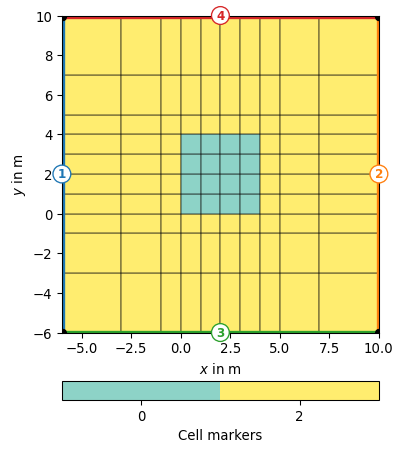Examples using pygimli.meshtools.appendTriangleBoundary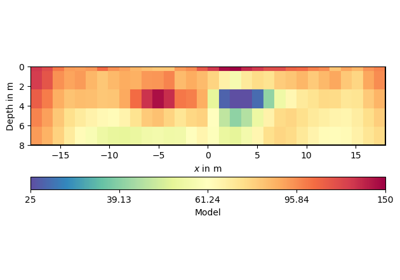### cellDataToBoundaryData¶

pygimli.meshtools.cellDataToBoundaryData(mesh, data)[source]

TODO DOCUMENT_ME

### cellDataToNodeData¶

pygimli.meshtools.cellDataToNodeData(mesh, data, style='mean')[source]

Convert cell data to node data.

Convert cell data to node data via non-weighted averaging (mean) of common cell data.

Parameters
• mesh (GIMLI::Mesh) – 2D or 3D GIMLi mesh

• data (iterable [float]) – Data of len mesh.cellCount(). TODO complex, R3Vector, ndarray

• style (str ['mean']) – Interpolation style. * ‘mean’ : non-weighted averaging TODO harmonic averaging TODO weighted averaging (mean, harmonic) TODO interpolation via cell centered mesh

Examples

>>> import pygimli as pg
>>> grid = pg.createGrid(x=(1,2,3),y=(1,2,3))
>>> celldata = np.array([1, 2, 3, 4])
>>> nodedata = pg.meshtools.cellDataToNodeData(grid, celldata)
>>> print(nodedata.array())
[1.  1.5 2.  2.  2.5 3.  3.  3.5 4. ]


Examples using pygimli.meshtools.cellDataToNodeData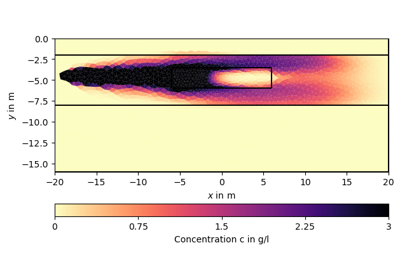### convert¶

pygimli.meshtools.convert(mesh, verbose=False)[source]

Convert mesh from foreign formats.

### convertHDF5Mesh¶

pygimli.meshtools.convertHDF5Mesh(h5Mesh, group='mesh', indices='cell_indices', pos='coordinates', cells='topology', marker='values', marker_default=0, dimension=3, verbose=True, useFenicsIndices=False)[source]

Converts instance of a hdf5 mesh to a GIMLI::Mesh.

For full documentation please see pygimli:meshtools:readHDF5Mesh.

### convertMeshioMesh¶

pygimli.meshtools.convertMeshioMesh(mesh, verbose=False)[source]

Convert mesh from meshio object.

TODO
• test for 3D mesh

• test and improve if neeeded

### createCircle¶

pygimli.meshtools.createCircle(pos=None, radius=1, nSegments=12, start=0, end=6.283185307179586, **kwargs)[source]

Create simple circle polygon.

Create simple circle polygon with given attributes.

Parameters
• pos ([x, y] [[0.0, 0.0]]) – Center position

• radius (float | [a,b] ) – radius or halfaxes of the circle

• nSegments (int ) – Discrete amount of segments for the circle.

• start (double ) – Starting angle in radians

• end (double [2*pi]) – Ending angle in radians

• **kwargs

marker: int 

Marker for the resulting triangle cells after mesh generation

markerPositionfloats [x, y] [0.0, 0.0]

Position of the marker (works for both regions and holes)

area: float 

Maximum cell size for resulting triangles after mesh generation

isHole: bool [False]

The polygon will become a hole instead of a triangulation

boundaryMarker: int 

Marker for the resulting boundary edges

leftDirection: bool [True]

Rotational direction

isClosed: bool [True]

Add closing edge between last and first node.

Returns

poly – The resulting polygon is a GIMLI::Mesh.

Return type

GIMLI::Mesh

>>>  # no need to import matplotlib. pygimli's show does
>>> import math
>>> from pygimli.viewer.mpl import drawMesh
>>> import pygimli.meshtools as mt
>>> c0 = mt.createCircle(pos=(-5.0, 0.0), radius=2, nSegments=6)
>>> c1 = mt.createCircle(pos=(-2.0, 2.0), radius=1, area=0.01, marker=2)
>>> c2 = mt.createCircle(pos=(0.0, 0.0), nSegments=5, start=0, end=math.pi)
>>> c3 = mt.createCircle(pos=(5.0, 0.0), nSegments=3, start=math.pi,
...                      end=1.5*math.pi, isClosed=False)
>>> plc = mt.mergePLC([c0, c1, c2, c3])
>>> fig, ax = pg.plt.subplots()
>>> drawMesh(ax, plc, fillRegion=False)
>>> pg.wait()


Examples using pygimli.meshtools.createCircle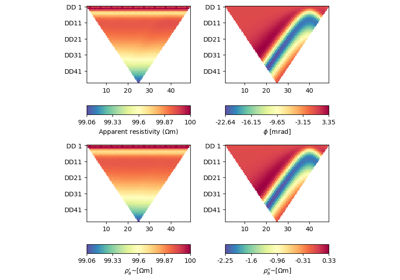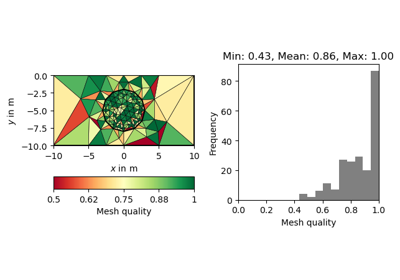### createCube¶

pygimli.meshtools.createCube(size=[1.0, 1.0, 1.0], pos=None, rot=None, boundaryMarker=0, **kwargs)[source]

Create cube PLC as geometrie definition.

Parameters
• size ([x, y, z]) – x, y, and z-size of the cube. Default = [1.0, 1.0, 1.0] in m

• pos (pg.Pos [None]) – The center position, default is at the origin.

• rot (pg.Pos [None]) – Rotate on the center.

• boundaryMarker (int) – Boundary marker for the resulting faces.

• kwargs (**) – Marker related arguments: See pygimli.meshtools.polytools.setPolyRegionMarker

Examples

>>> import pygimli.meshtools as mt
>>> cube = mt.createCube()
>>> print(cube)
Mesh: Nodes: 8 Cells: 0 Boundaries: 6
>>> cube = mt.createCube([10, 10, 1])
>>> print(cube.bb())
[[-5.0, -5.0, -0.5], [5.0, 5.0, 0.5]]
>>> cube = mt.createCube([10, 10, 1], pos=[-4.0, 0.0, 0.0])
>>> print(pg.center(cube.positions()))
RVector3: (-4.0, 0.0, 0.0)

Returns

poly – The resulting polygon is a GIMLI::Mesh.

Return type

GIMLI::Mesh

Examples using pygimli.meshtools.createCube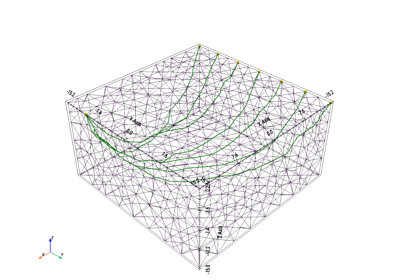### createCylinder¶

pygimli.meshtools.createCylinder(radius=1, height=1, nSegments=8, pos=None, rot=None, boundaryMarker=0, **kwargs)[source]

Create PLC of a cylinder.

Out of core wrapper for dcfemlib::polytools.

Note, there is a bug in the old polytools which ignores the area settings for marker == 0.

Parameters
• radius (float) – Radius of the cylinder.

• height (float) – Height of the cylinder

• nSegments (int ) – Number of segments of the cylinder.

• pos (pg.Pos [None]) – The center position, default is at the origin.

Keyword Arguments

kwargs (**) – Marker related arguments: See pygimli.meshtools.polytools.setPolyRegionMarker

Returns

poly – The resulting polygon is a GIMLI::Mesh.

Return type

GIMLI::Mesh

### createFacet¶

pygimli.meshtools.createFacet(mesh, boundaryMarker=None, verbose=True)[source]

Create a coplanar PLC of a 2d mesh or poly

TODO: * mesh with cell into plc with boundaries * poly account for inner edges

### createGrid¶

pygimli.meshtools.createGrid(x=None, y=None, z=None, **kwargs)[source]

Create grid style mesh.

Generate simple grid with defined node positions for each dimension. The resulting grid depends on the amount of given coordinate arguments and consists out of edges (1D - x), quads (2D- x and y), or hexahedrons(3D- x, y, and z).

Parameters

kwargs

x: array

x-coordinates for all Nodes (1D, 2D, 3D)

y: array

y-coordinates for all Nodes (2D, 3D)

z: array

z-coordinates for all Nodes (3D)

markerint = 0

Marker for resulting cells.

worldBoundaryMarkerbool = False

Boundaries are enumerated with world marker, i.e., Top = -1 All remaining = -2. Default marker are left=1, right=2, top=3, bottom=4, front=5, back=6

>>> import pygimli as pg
>>> mesh = pg.meshtools.createGrid(x=[0, 1, 1.5, 2], y=[-1, -0.5, -0.25, 0],
...                                 marker=2)
>>> print(mesh)
Mesh: Nodes: 16 Cells: 9 Boundaries: 24
>>> fig, axs = pg.plt.subplots(1, 2)
>>> _ = pg.show(mesh, markers=True, showMesh=True, ax=axs)
>>> mesh = pg.meshtools.createGrid(x=[0, 1, 1.5, 2], y=[-1, -0.5, -0.25, 0],
...                                worldBoundaryMarker=True, marker=2)
>>> print(mesh)
Mesh: Nodes: 16 Cells: 9 Boundaries: 24
>>> _ = pg.show(mesh, markers=True, showBoundaries=True,
...             showMesh=True, ax=axs)


(png, pdf)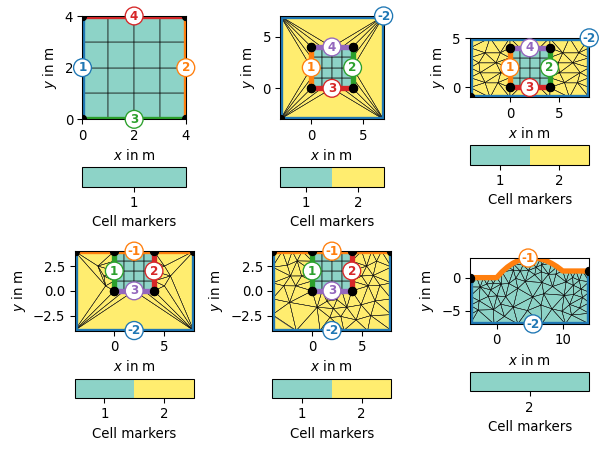### createGridPieShaped¶

pygimli.meshtools.createGridPieShaped(x, degree=10.0, h=2, marker=0)[source]

Create a 2D pie shaped grid (segment from annulus or cirlce).

• degree: > 90 .. 360

Parameters
• x (array) – x-coordinates for all Nodes (2D). If you need it 3D, you can apply pygimli.meshtools.extrudeMesh on it.

• degree (float [None]) – Create a pie shaped grid for a value between 0 and 90. Creates an optional inner boundary (marker=2) for a annulus with x > 0. Outer boundary marker is 1. Optional h refinement. Center node is the first for circle segment.

• h (int ) – H-Refinement for degree option.

• marker (int = 0) – Marker for resulting cells.

Returns

mesh

Return type

GIMLI::Mesh

>>> import pygimli as pg
>>> mesh = pg.meshtools.createGridPieShaped(x=[0, 1, 3], degree=45, h=3)
>>> print(mesh)
Mesh: Nodes: 117 Cells: 128 Boundaries: 244
>>> _ = pg.show(mesh)
>>> mesh = pg.meshtools.createGridPieShaped(x=[1, 2, 3], degree=45, h=3)
>>> print(mesh)
Mesh: Nodes: 153 Cells: 128 Boundaries: 280
>>> _ = pg.show(mesh)(png, pdf)(png, pdf)

### createLine¶

pygimli.meshtools.createLine(start, end, nSegments=1, **kwargs)[source]

Create simple line polygon.

Create simple line polygon from start to end.

Parameters
• start ([x, y]) – start position

• end ([x, y]) – end position

• nSegments (int) – Discrete amount of segments for the line

Keyword Arguments
• boundaryMarker (int ) – Marker for the resulting boundary edges

• leftDirection (bool [True]) – Rotational direction

Returns

poly – The resulting polygon is a GIMLI::Mesh.

Return type

GIMLI::Mesh

>>>  # no need to import matplotlib. pygimli's show does
>>> import pygimli as pg
>>> import pygimli.meshtools as mt
>>>
>>> w = mt.createWorld(start=[0, 0], end=[3, 3])
>>> l1 = mt.createLine(start=[1, 1], end=[1, 2], nSegments=1,
...                    leftDirection=False)
>>> l2 = mt.createLine(start=[1, 1], end=[2, 1], nSegments=20,
...                    leftDirection=True)
>>>
>>> ax, _ = pg.show(mt.createMesh([w, l1, l2,]))
>>> ax, _ = pg.show([w, l1, l2,], ax=ax, fillRegion=False)
>>> pg.wait()


(png, pdf)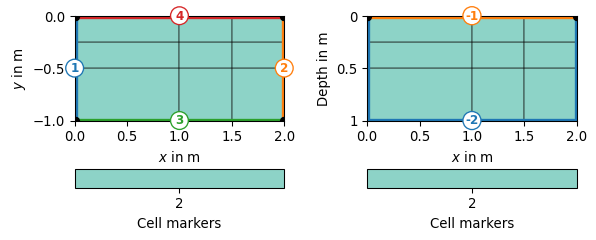### createMesh¶

pygimli.meshtools.createMesh(poly, quality=32, area=0.0, smooth=None, switches=None, verbose=False, **kwargs)[source]

Create a mesh for a given PLC or point list.

The mesh is created by triangle or tetgen if the pgGIMLi support for these mesh generators is installed. A PLC needs to contain nodes and boundaries and should be valid in the sense that the boundaries are non-intersecting.

If poly is a list of coordinates, a simple Delaunay mesh with a convex hull will be created. Quality and area arguments are ignored for this case to create a mesh with one node for each coordinate position.

Parameters
• poly (GIMLI::Mesh or list or ndarray) –

• 2D or 3D gimli mesh that contains the PLC.

• 2D mesh needs edges

• 3D mesh needs a plc and tetgen as system component

• List of x y pairs [[x0, y0], … ,[xN, yN]]

• ndarray [x_i, y_i]

• PLC or list of PLCs

• quality (float) – 2D triangle quality sets a minimum angle constraint. Be careful with values above 34 degrees. 3D tetgen quality. Be careful with values below 1.12.

• area (float) – Maximum element size (global). 2D maximum triangle size in m*², 3D maximum tetrahedral size in m³.

• smooth (tuple) –

[smoothing algorithm, number of iterations] 0: no smoothing 1: node center 2: weighted node center

If smooth is just set to True then [1, 4] is choosen.

• switches (str) – Set additional triangle command switches. https://www.cs.cmu.edu/~quake/triangle.switch.html

Returns

mesh

Return type

GIMLI::Mesh

>>> import pygimli as pg
>>> import pygimli.meshtools as mt
>>> rect = mt.createRectangle(start=[0, 0], end=[4, 1])
>>> ax, _ = pg.show(mt.createMesh(rect, quality=10))
>>> ax, _ = pg.show(mt.createMesh(rect, quality=33))
>>> ax, _ = pg.show(mt.createMesh(rect, quality=33, area=0.01))
>>> pg.wait()(png, pdf)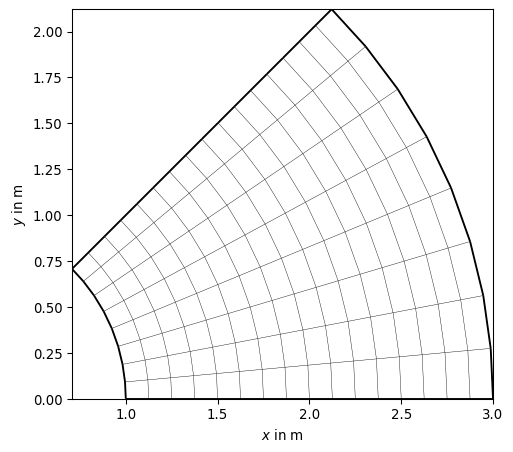(png, pdf)(png, pdf)

Examples using pygimli.meshtools.createMesh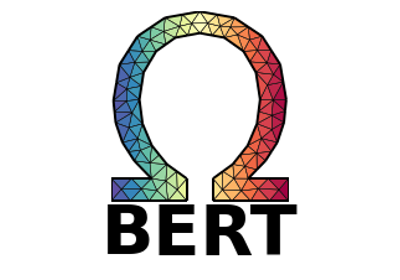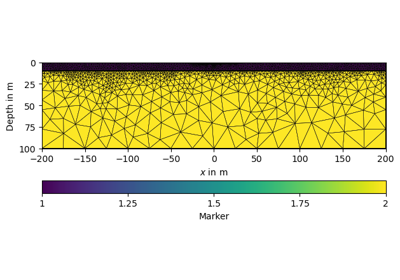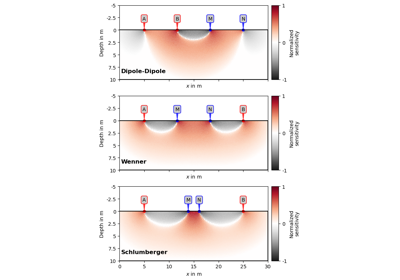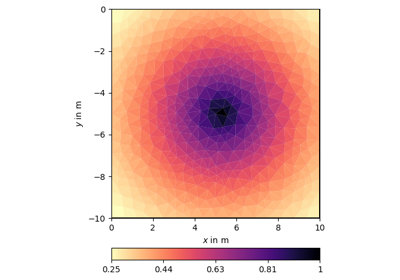### createMesh1D¶

pygimli.meshtools.createMesh1D((object)x) → object :

Generate simple one dimensional mesh with nodes at position in RVector pos.

C++ signature :

GIMLI::Mesh createMesh1D(GIMLI::Vector<double>)

createMesh1D( (object)nCells [, (object)nProperties=1]) -> object :

Generate simple 1D mesh with nCells cells of length 1, and nCells + 1 nodes. In case of more than one property quasi-2d mesh with regions is generated.

C++ signature :

GIMLI::Mesh createMesh1D(unsigned long [,unsigned long=1])

### createMesh1DBlock¶

pygimli.meshtools.createMesh1DBlock((object)nLayers[, (object)nProperties=1]) → object :

Generate 1D block model of thicknesses and properties

C++ signature :

GIMLI::Mesh createMesh1DBlock(unsigned long [,unsigned long=1])

Examples using pygimli.meshtools.createMesh1DBlock### createMesh2D¶

pygimli.meshtools.createMesh2D((object)x, (object)y[, (object)markerType=0]) → object :

Generate simple two dimensional mesh with nodes at position in RVector x and y.

C++ signature :

GIMLI::Mesh createMesh2D(GIMLI::Vector<double>,GIMLI::Vector<double> [,int=0])

createMesh2D( (object)mesh, (object)y [, (object)frontMarker=0 [, (object)backMarker=0 [, (object)leftMarker=0 [, (object)rightMarker=0 [, (object)adjustBack=False]]]]]) -> object :

Generate a simple 2D mesh by extruding a 1D polygone into RVector y using quads. We assume a 2D mesh here consisting on nodes and edge boundaries. Nodes with marker are extruded as edges with marker or set to front- and backMarker. Edges with marker are extruded as cells with marker. All back y-coordinates are adjusted if adjustBack is set.

C++ signature :

GIMLI::Mesh createMesh2D(GIMLI::Mesh,GIMLI::Vector<double> [,int=0 [,int=0 [,int=0 [,int=0 [,bool=False]]]]])

createMesh2D( (object)xDim, (object)yDim [, (object)markerType=0]) -> object :

Generate simple two dimensional mesh with nRows x nCols cells with each length = 1.0

C++ signature :

GIMLI::Mesh createMesh2D(unsigned long,unsigned long [,int=0])

Examples using pygimli.meshtools.createMesh2D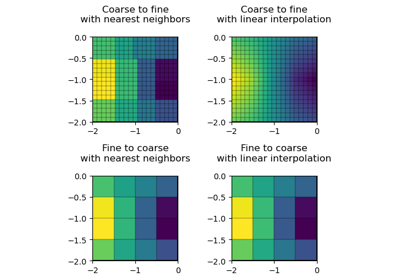### createMesh3D¶

pygimli.meshtools.createMesh3D((object)x, (object)y, (object)z[, (object)markerType=0]) → object :

Generate simple three dimensional mesh with nodes at position in RVector x and y.

C++ signature :

GIMLI::Mesh createMesh3D(GIMLI::Vector<double>,GIMLI::Vector<double>,GIMLI::Vector<double> [,int=0])

createMesh3D( (object)mesh, (object)z [, (object)topMarker=0 [, (object)bottomMarker=0]]) -> object :

Generate a simple three dimensional mesh by extruding a two dimensional mesh into RVector z using triangle prism or hexahedrons or both. 3D cell marker are set from 2D cell marker. The boundary marker for the side boundaries are set from edge marker in mesh. Top and bottomLayer boundary marker are set from parameter topMarker and bottomMarker.

C++ signature :

GIMLI::Mesh createMesh3D(GIMLI::Mesh,GIMLI::Vector<double> [,int=0 [,int=0]])

createMesh3D( (object)xDim, (object)yDim, (object)zDim [, (object)markerType=0]) -> object :

Generate simple three dimensional mesh with nx x nx x nz cells with each length = 1.0

C++ signature :

GIMLI::Mesh createMesh3D(unsigned long,unsigned long,unsigned long [,int=0])

### createMeshFromHull¶

pygimli.meshtools.createMeshFromHull(mesh, fixNodes=[], **kwargs)[source]

Create a new 2D triangular mesh from the boundaries of mesh.

Parameters
• mesh (GIMLI::Mesh) – Input mesh.

• fixNodes (iterable (int)) – Nodes (IDs) from the input mesh that should be part of the new mesh.

Keyword Arguments

**kwargs (dict) – Forwarded to pygimli:meshtools:createMesh.

Returns

mesh – Returning mesh. If fixed nodes are requested, a list of the new IDs are returned in advance.

Return type

GIMLI::Mesh, List of fixed nodes

### createParaDomain2D¶

pygimli.meshtools.createParaDomain2D(*args, **kwargs)[source]

API change here .. use createParaMeshPLC instead.

### createParaMesh¶

pygimli.meshtools.createParaMesh(*args, **kwargs)[source]

Create parameter mesh from list of sensor positions.

Create parameter mesh from list of sensor positions.

Parameters
• sensors (list of RVector3 objects) – Sensor positions. Must be sorted and unique in positive x direction. Depth need to be y-coordinate.

• paraDX (float) – Relative distance for refinement nodes between two electrodes (1=none), e.g., 0.5 means 1 additional node between two neighboring electrodes e.g., 0.33 means 2 additional equidistant nodes between two electrodes

• paraDepth (float, optional) – Maximum depth for parametric domain, 0 (default) means 0.4 * maximum sensor range.

• paraBoundary (float, optional) – Margin for parameter domain in absolute sensor distances. 2 (default).

• paraMaxCellSize (double , optional) – Maximum size for parametric size in m*m (0-no constraint)

• boundaryMaxCellSize (double , optional) – Maximum cells size in the boundary region in m*m (0-no constraint)

• boundary (float, optional) – Boundary width to be appended for domain prolongation in absolute para domain width. Values <=0 force the boundary to be 4 times para domain width.

Returns

poly

Return type

GIMLI::Mesh

Examples using pygimli.meshtools.createParaMesh### createParaMesh2DGrid¶

pygimli.meshtools.createParaMesh2DGrid(sensors, paraDX=1, paraDZ=1, paraDepth=0, nLayers=11, boundary=- 1, paraBoundary=2, **kwargs)[source]

Create a grid-style mesh for an inversion parameter mesh.

Create a grid-style mesh for an inversion parameter mesh. Return parameter grid for a given list of sensor positions. Uses and forwards arguments to pygimli.meshtools.appendTriangleBoundary.

Parameters
• sensors (list of RVector3 objects or data container with sensorPositions) – Sensor positions. Must be sorted in positive x direction

• paraDX (float, optional) – Horizontal distance between sensors, relative regarding sensor distance. Value must be greater than 0 otherwise 1 is assumed.

• paraDZ (float, optional) – Vertical distance to the first depth layer, relative regarding sensor distance. Value must be greater than 0 otherwise 1 is assumed.

• paraDepth (float, optional) – Maximum depth for parametric domain, 0 (default) means 0.4 * maximum sensor range.

• nLayers (int, optional ) – Number of depth layers.

• boundary (int, optional [-1]) – Boundary width to be appended for domain prolongation in absolute para domain width. Values lower than 0 force the boundary to be 4 times para domain width.

• paraBoundary (int, optional ) – Offset to the parameter domain boundary in absolute sensor spacing.

Returns

mesh

Return type

GIMLI::Mesh

>>> import pygimli as pg
>>> import matplotlib.pyplot as plt
>>>
>>> from pygimli.meshtools import createParaMesh2DGrid
>>> mesh = createParaMesh2DGrid(sensors=pg.Vector(range(10)),
...                             boundary=1, paraDX=1,
...                             paraDZ=1, paraDepth=5)
>>> ax, _ = pg.show(mesh, markers=True, showMesh=True)


(png, pdf)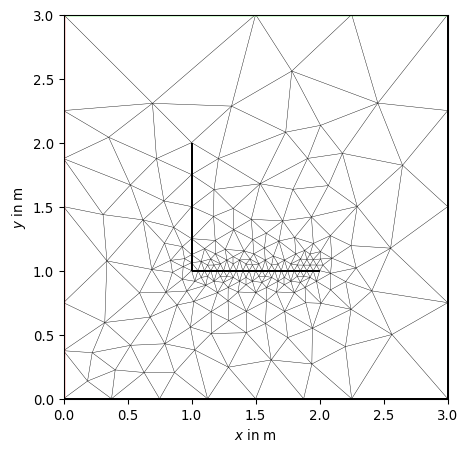### createParaMeshPLC¶

pygimli.meshtools.createParaMeshPLC(sensors, paraDX=1, paraDepth=0, paraBoundary=2, paraMaxCellSize=0.0, boundary=- 1, boundaryMaxCellSize=0, balanceDepth=True, isClosed=False, addNodes=1, **kwargs)[source]

Create a PLC mesh for an inversion parameter mesh.

Create a PLC mesh for an inversion parameter mesh with for a given list of sensor positions. Sensor positions are assumed to be on the surface and must be sorted and unique.

You can create a parameter mesh without sensors if you just set [xMin, xMax] as sensors.

The PLC is a GIMLI::Mesh and contain nodes, edges and two region markers, one for the parameters domain (marker=2) and a larger boundary around the outside (marker=1)

Parameters
• sensors ([RVector3] | DataContainer with sensorPositions() | [xMin, xMax]) – Sensor positions. Must be sorted and unique in positive x direction. Depth need to be y-coordinate.

• paraDX (float ) – Relative distance for refinement nodes between two sensors (1=none), e.g., 0.5 means 1 additional node between two neighboring sensors e.g., 0.33 means 2 additional equidistant nodes between two sensors

• paraDepth (float, optional) – Maximum depth for parametric domain, 0 (default) means 0.4 * maximum sensor range.

• balanceDepth (bool [True]) – Equal depth for the parametric domain.

• paraBoundary (float, optional) – Margin for parameter domain in absolute sensor distances. 2 (default).

• paraMaxCellSize (double, optional) – Maximum size for parametric size in m*m

• boundaryMaxCellSize (double, optional) – Maximum cells size in the boundary region in m*m

• boundary (float, optional) – Boundary width to be appended for domain prolongation in absolute para domain width. Values lover 0 force the boundary to be 4 times para domain width.

• isClosed (bool [False]) – Create a closed geometry from sensor positions. Region marker is 1. Boundary marker is -1 (homogeneous Neumann)

• addNodes (int ) – Number of additional nodes to be added equidistant between sensors.

Returns

poly – piecewise linear complex (PLC) containing nodes and edges

Return type

GIMLI::Mesh

>>> # no need to import matplotlib, pygimli show does.
>>> import pygimli as pg
>>> import pygimli.meshtools as plc
>>> # Create the simplest paramesh PLC with a para box of 10 m without
>>> # sensors
>>> p = plc.createParaMeshPLC([0,10])
>>> # you can add subsurface sensors now with
>>> for z in range(1,4):
...     n = p.createNode((5,-z), -99)
>>> ax,_ = pg.show(p)


(png, pdf)### createPolygon¶

pygimli.meshtools.createPolygon(verts, isClosed=False, addNodes=0, interpolate='linear', **kwargs)[source]

Create a polygon from a list of vertices.

All vertices need to be unique and duplicate vertices will be ignored. If you want the polygon be a closed region you can set the ‘isClosed’ flag. Closed region can be attributed by assigning a region marker. The automatic region marker is placed in the center of all vertices.

Parameters
• verts ([]) –

• List of x y pairs [[x0, y0], … ,[xN, yN]]

• isClosed (bool [True]) – Add closing edge between last and first node.

• addNodes (int ) – Number of additional nodes to be added equidistant between sensors.

• interpolate (str ['linear']) – Interpolation rule for addNodes. ‘linear’ or ‘spline’. TODO ‘harmfit’

• **kwargs

markerint [None]

Marker for the resulting triangle cells after mesh generation.

markerPositionfloats [x, y] [0.0, 0.0]

Position (absolute) of the marker (works for both regions and holes)

areafloat 

Maximum cell size for resulting triangles after mesh generation

isHolebool [False]

The polygon will become a hole instead of a triangulation

boundaryMarkerint 

Marker for the resulting boundary edges

leftDirectionbool [True]

Rotational direction

Returns

poly – The resulting polygon is a GIMLI::Mesh.

Return type

GIMLI::Mesh

>>> # no need to import matplotlib, pygimli show does.
>>> import pygimli as pg
>>> import pygimli.meshtools as mt
>>> p1 = mt.createPolygon([[0.0, 0.0], [1.0, 0.0], [1.0, 1.0]],
...                       isClosed=True, marker=3, area=0.1)
>>> p2 = mt.createPolygon([[0.3, 0.15], [0.85, 0.15], [0.85, 0.7]],
...                       isClosed=True, isHole=True)
>>> p3 = mt.createPolygon([[-0.1, 0.2], [-1.1, 0.2], [-1.1, 1.2], [-0.1, 1.2]],
...                       isClosed=True, addNodes=3, marker=2)
>>> p4 = mt.createPolygon([[-0.1, 0.2], [-1.1, 0.2], [-1.1, 1.2], [-0.1, 1.2]],
...                       isClosed=True, addNodes=5, interpolate='spline',
...                       marker=4)
>>> ax, _ = pg.show(mt.mergePLC([p1, p2, p3, p4]), showNodes=True)
>>> pg.wait()


(png, pdf)Examples using pygimli.meshtools.createPolygon### createRectangle¶

pygimli.meshtools.createRectangle(start=None, end=None, pos=None, size=None, **kwargs)[source]

Create rectangle polygon.

Create rectangle with start position and a given size. Give either start and end OR pos and size.

Parameters
• start ([x, y]) – Left upper corner. Default [-0.5, 0.5]

• end ([x, y]) – Right lower corner. Default [0.5, -0.5]

• pos ([x, y]) – Center position. The rectangle will be moved.

• size ([x, y]) – Factors for x and y by which the rectangle, defined by start and width, are scaled.

Keyword Arguments

**kwargs

Additional kwargs

markerint 

Marker for the resulting triangle cells after mesh generation

markerPositionfloats [x, y] [pos + (end - start) * 0.2]

Absolute position of the marker (works for both regions and holes).

areafloat 

Maximum cell size for resulting triangles after mesh generation

isHolebool [False]

The polygon will become a hole instead of a triangulation

boundaryMarkerint 

Marker for the resulting boundary edges

leftDirectionbool [True]

TODO Rotational direction

Returns

poly – The resulting polygon is a GIMLI::Mesh.

Return type

GIMLI::Mesh

>>> # no need to import matplotlib, pygimli show does.
>>> import pygimli as pg
>>> import pygimli.meshtools as mt
>>> r1 = mt.createRectangle(pos=[1, -1], size=[4.0, 4.0],
...                      marker=1, area=0.1, markerPosition=[0, -2])
>>> r2 = mt.createRectangle(start=[0.5, -0.5], end=[2, -2],
...                      marker=2, area=1.1)
>>> _ = pg.show(mt.mergePLC([r1, r2]))
>>> pg.wait()


(png, pdf)Examples using pygimli.meshtools.createRectangle### createWorld¶

pygimli.meshtools.createWorld(start, end, marker=1, area=0.0, layers=None, worldMarker=True, **kwargs)[source]

Create simple rectangular world.

Create simple rectangular [hexagonal] world with appropriate boundary conditions. Surface boundary is set do pg.core.MARKER_BOUND_HOMOGEN_NEUMANN, i.e, -1 and inner subsurface is set to pg.core.MARKER_BOUND_MIXED, i.e., -2 or Numbered in ascending order in left direction starting upper left if worldMarker is set to false.

Parameters
• start ([x, y, [z]]) – Upper/Left/[Front] Corner

• end ([x, y, [z]]) – Lower/Right/[Back] Corner

• marker (int) – Marker for the resulting triangle cells after mesh generation.

• area (float | list) – Maximum cell size for resulting triangles after mesh generation. If area is a float set it global, if area is a list set it per layer.

• layers ([float] [None]) – List of depth coordinates for some layers.

• worldMarker (bool [True]) – Specify kind of preset boundary marker [-1, -2] or ascending order [1, 2, 3, 4 ..]

Other Parameters

Forwarded to createCube

Returns

poly – The resulting polygon is a GIMLI::Mesh.

Return type

GIMLI::Mesh

>>> from pygimli.meshtools import createWorld
>>> from pygimli.viewer.mpl import drawMesh
>>> import matplotlib.pyplot as plt
>>> world = createWorld(start=[-5, 0], end=[5, -5], layers=[-1,-2,-3])
>>>
>>> fig, ax = plt.subplots()
>>> drawMesh(ax, world)
>>> plt.show()


(png, pdf)Examples using pygimli.meshtools.createWorld### exportFenicsHDF5Mesh¶

pygimli.meshtools.exportFenicsHDF5Mesh(mesh, exportname)[source]

Exports Gimli mesh in HDF5 format suitable for Fenics.

Equivalent to calling the function pygimli.meshtools.exportHDF5Mesh(mesh, exportname, group=['mesh', 'domains'], indices='cell_indices', pos='coordinates', cells='topology', marker='values').

Parameters
• mesh (:gimliapi:GIMLI::Mesh) – Mesh to be saved.

• exportname (string) – Name under which the mesh is saved.

### exportHDF5Mesh¶

pygimli.meshtools.exportHDF5Mesh(mesh, exportname, group='mesh', indices='cell_indices', pos='coordinates', cells='topology', marker='values')[source]

Writes given GIMLI::Mesh in a hdf5 format file.

3D tetrahedral meshes only! Boundary markers are ignored.

Keywords are explained in pygimli.meshtools.readHDFS

### exportPLC¶

pygimli.meshtools.exportPLC(poly, fname, **kwargs)[source]

General writer to save a piece-wise linear complex (PLC), i.e. a pyGIMLi geometry, as a poly file.

Choose from poly.dimension() and forward appropriate to GIMLI::Mesh::exportAsTetgenPolyFile and pygimli.meshtools.writeTrianglePoly

Parameters
• poly (GIMLI::Mesh) – The polygon to be written.

• fname (string) – Filename of the file to write (\*.n, \*.e).

Examples

>>> import pygimli as pg
>>> import tempfile, os
>>> fname = tempfile.mktemp() + '.poly' # Create temporary filename.
>>> world2d = pg.meshtools.createWorld(start=[-10, 0], end=[20, -10])
>>> pg.meshtools.exportPLC(world2d, fname)
>>> read2d = pg.meshtools.readPLC(fname)
>>> print(read2d)
Mesh: Nodes: 4 Cells: 0 Boundaries: 4
>>> world3d = pg.createGrid([0, 1], [0, 1], [-1, 0])
>>> pg.meshtools.exportPLC(world3d, fname)
>>> os.remove(fname)


### exportSTL¶

pygimli.meshtools.exportSTL(mesh, fileName, binary=False)[source]

Write STL surface mesh and returns a GIMLI::Mesh.

Export a three dimensional boundary GIMLI::Mesh into a STL surface mesh. Boundaries with different marker will be separated into different STL solids.

Parameters
• mesh (GIMLI::Mesh) – Mesh to be exported. Only Boundaries of type TriangleFace will be exported.

• fileName (str) – name of the .stl file containing the STL surface mesh

• binary (bool [False]) – Write STL binary format. TODO

### extrude¶

pygimli.meshtools.extrude(p2, z=- 1.0, boundaryMarker=0, **kwargs)[source]

Create 3D body by extruding a closed 2D poly into z direction

Parameters
Keyword Arguments

kwargs (**) – Marker related arguments: See pygimli.meshtools.polytools.setPolyRegionMarker

Returns

poly – The resulting polygon is a GIMLI::Mesh.

Return type

GIMLI::Mesh

### extrudeMesh¶

pygimli.meshtools.extrudeMesh(mesh, a, **kwargs)[source]

Extrude mesh to a higher dimension.

Generates a 2D mesh by extruding a 1D mesh along y-coordinate using quads. We assume a 2D mesh here consisting of nodes and edges. The marker of nodes are extruded as edges with the same marker. The marker of the edges are extruded as cells with same marker. Optionally all y-coordinates can be adjusted to become equal at the end

Generates a three-dimensional mesh by extruding a two-dimensional mesh along the z-coordinate transforming triangles into triangular prisms or quads into hexahedrons. 3D cell markers are set from 2D cell marker. The boundary marker for the side boundaries are set from edge markers.

Parameters
• mesh (GIMLI::Mesh) – Input mesh

• a (iterable (float)) – Additional coordinate to extrude into.

Keyword Arguments

adjustBottom (bool [False]) – Adjust all nodes such that the bottom of the mesh has a constant depth (only 2D)

Returns

mesh – Returning mesh of +1 dimension

Return type

GIMLI::Mesh

>>> import numpy as np
>>> import pygimli as pg
>>> import pygimli.meshtools as mt
>>> topo = [[x, 1.0 + np.cos(2 * np.pi * 1/30 * x)] for x in range(31)]
>>> m1 = mt.createPolygon(topo)
>>> m1.setBoundaryMarkers(range(m1.boundaryCount()))

>>> m = mt.extrudeMesh(m1, a=-(np.geomspace(1, 5, 8)-1.0))
>>> _ = pg.show(m, m.cellMarkers(), showMesh=True)
>>> m = mt.extrudeMesh(m1, a=-(np.geomspace(1, 5, 8)-1.0),
...                    adjustBottom=True)
>>> _ = pg.show(m, m.cellMarkers(), showMesh=True)(png, pdf)(png, pdf)

Examples using pygimli.meshtools.extrudeMesh### fillEmptyToCellArray¶

pygimli.meshtools.fillEmptyToCellArray(mesh, vals, slope=True)[source]

Prolongate empty cell values to complete cell attributes.

It is possible to have zero values that are filled with appropriate attributes. This function tries to fill empty values successively by prolongation of the non-zeros.

Parameters
• mesh (GIMLI::Mesh) – For each cell of mesh a value will be returned.

• vals (array) – Array of size cellCount().

Returns

atts – Array of length mesh.cellCount()

Return type

array

>>> import pygimli as pg
>>> import numpy as np
>>> import matplotlib.pyplot as plt
>>>
>>> # Create a mesh with 3 layers and an outer region for extrapolation
>>> layers = pg.meshtools.createWorld([0,-50],[100,0], layers=[-15,-35])
>>> inner = pg.meshtools.createMesh(layers, area=3)
>>> mesh = pg.meshtools.appendTriangleBoundary(inner, xbound=120, ybound=50,
...                                            area=20, marker=0)
>>>
>>> # Create data for the inner region only
>>> layer_vals = [20,30,50]
>>> data = np.array(layer_vals)[inner.cellMarkers() - 1]
>>>
>>> # The following fails since len(data) != mesh.cellCount(), extrapolate
>>> # pg.show(mesh, data)
>>>
>>> # Create data vector, where zeros fill the outer region
>>> data_with_outer = np.array( + layer_vals)[mesh.cellMarkers()]
>>>
>>> # Actual extrapolation
>>> extrapolated_data = pg.meshtools.fillEmptyToCellArray(mesh,
...                                  data_with_outer, slope=False)
>>> extrapolated_data_with_slope = pg.meshtools.fillEmptyToCellArray(mesh,
...                                 data_with_outer, slope=True)
>>>
>>> # Visualization
>>> fig, (ax1, ax2, ax3) = plt.subplots(1,3, figsize=(10,8), sharey=True)
>>> _ = pg.show(mesh, data_with_outer, ax=ax1, cMin=0)
>>> _ = pg.show(mesh, extrapolated_data, ax=ax2, cMin=0)
>>> _ = pg.show(mesh, extrapolated_data_with_slope, ax=ax3, cMin=0)
>>> _ = ax1.set_title("Original data")
>>> _ = ax2.set_title("Extrapolated with slope=False")
>>> _ = ax3.set_title("Extrapolated with slope=True")


(png, pdf)### interpolate¶

pygimli.meshtools.interpolate(*args, **kwargs)[source]

Interpolation convinience function.

Convenience function to interpolate different kind of data. Currently supported interpolation schemes are:

• Interpolate mesh based data from one mesh to another

(syntactic sugar for the core based interpolate (see below))

Parameters:
args: GIMLI::Mesh, GIMLI::Mesh, iterable

outData = interpolate(outMesh, inMesh, vals) Interpolate values based on inMesh to outMesh. Values can be of length inMesh.cellCount() interpolated to outMesh.cellCenters() or inMesh.nodeCount() which are interpolated to outMesh.positions().

Returns:

Interpolated values.

• Mesh based values to arbitrary points, based on finite element interpolation (from gimli core).

Parameters:
args: GIMLI::Mesh, …

Arguments forwarded to GIMLI::interpolate()

kwargs:

Arguments forwarded to GIMLI::interpolate()

interpolate(srcMesh, destMesh)

All data from inMesh are interpolated to outMesh

Returns:

Interpolated values

• Interpolate along curve. Forwarded to pygimli.meshtools.interpolateAlongCurve

Parameters:

args: curve, t

kwargs:

Arguments forwarded to pygimli.meshtools.interpolateAlongCurve

periodicbool [False]

Curve is periodic. Useful for closed parametric spline interpolation.

• 1D point set $$u(x)$$ for ascending $$x$$. Find interpolation function $$I = u(x)$$ and returns $$u_{\text{i}} = I(x_{\text{i}})$$ (interpolation methods are [linear via matplotlib, cubic spline via scipy, fit harmonic functions’ via pygimli]) Note, for ‘linear’ and ‘spline’ the interpolate contains all original coordinates while ‘harmonic’ returns an approximate best fit. The amount of harmonic coefficients can be specfied with the ‘nc’ keyword.

Parameters:
args: xi, x, u
• $$x_{\text{i}}$$ - target sample points

• $$x$$ - function sample points

• $$u$$ - function values

kwargs:
• methodstring

Specify interpolation method ‘linear, ‘spline’, ‘harmonic’

• ncint

Number of harmonic coefficients for the ‘harmonic’ method.

• periodicbool [False]

Curve is periodic. Useful for closed parametric spline interpolation.

Returns:
ui: array of length xi

$$u_{\text{i}} = I(x_{\text{i}})$$, with $$I = u(x)$$

To use the core functions GIMLI::interpolate() start with a mesh instance as first argument or use the appropriate keyword arguments.

TODO

• 2D parametric to points (method=[‘linear, ‘spline’, ‘harmonic’])

• 2D/3D point cloud to points/grids

(‘Delauney’, ‘linear, ‘spline’, ‘harmonic’)

• Mesh to points based on nearest neighbor values (pg.core)

>>> import numpy as np
>>> import pygimli as pg
>>> fig, ax = pg.plt.subplots(1, 1, figsize=(10, 5))
>>> u = np.array([1.0, 12.0, 3.0, -4.0, 5.0, 6.0, -1.0])
>>> xu = np.array(range(len(u)))
>>> xi = np.linspace(xu, xu[-1], 1000)
>>> _= ax.plot(xu, u, 'o')
>>> _= ax.plot(xi, pg.interpolate(xi, xu, u, method='linear'),
...         color='blue', label='linear')
>>> _= ax.plot(xi, pg.interpolate(xi, xu, u, method='spline'),
...            color='red', label='spline')
>>> _= ax.plot(xi, pg.interpolate(xi, xu, u, method='harmonic'),
...         color='green', label='harmonic')
>>> _= ax.legend()
>>>
>>> pg.plt.show()


(png, pdf)### interpolateAlongCurve¶

pygimli.meshtools.interpolateAlongCurve(curve, t, **kwargs)[source]

Interpolate along curve.

Return curve coordinates for a piecewise linear curve $$C(t) = {x_i,y_i,z_i}$$ at positions $$t$$. Curve and $$t$$ values are expected to be sorted along distance from the origin of the curve.

Parameters
• curve ([[x,z]] | [[x,y,z]] | [GIMLI::RVector3] | GIMLI::R3Vector) – Discrete curve for 2D $$x,z$$ curve=[[x,z]], 3D $$x,y,z$$

• t (1D iterable) – Query positions along the curve in absolute distance

• kwargs

If kwargs are given, an additional curve smoothing is applied using pygimli.meshtools.interpolate. The kwargs will be delegated.

periodicbool [False]

Curve is periodic. Usefull for closed parametric spline interpolation.

Returns

p – Curve positions at query points $$t$$. Dimension of p match the size of curve the coordinates.

Return type

np.array

>>> import numpy as np
>>> import pygimli as pg
>>> import pygimli.meshtools as mt
>>> fig, axs = pg.plt.subplots(2,2)
>>> topo = np.array([[-2., 0.], [-1., 0.], [0.5, 0.], [3., 2.], [4., 2.], [6., 1.], [10., 1.], [12., 1.]])
>>> t = np.arange(15.0)
>>> p = mt.interpolateAlongCurve(topo, t)
>>> _= axs[0,0].plot(topo[:,0], topo[:,1], '-x', mew=2)
>>> _= axs[0,1].plot(p[:,0], p[:,1], 'o', color='red')
>>>
>>> p = mt.interpolateAlongCurve(topo, t, method='spline')
>>> _= axs[1,0].plot(p[:,0], p[:,1], '-o', color='black')
>>>
>>> p = mt.interpolateAlongCurve(topo, t, method='harmonic', nc=3)
>>> _= axs[1,1].plot(p[:,0], p[:,1], '-o', color='green')
>>>
>>> pg.plt.show()


(png, pdf)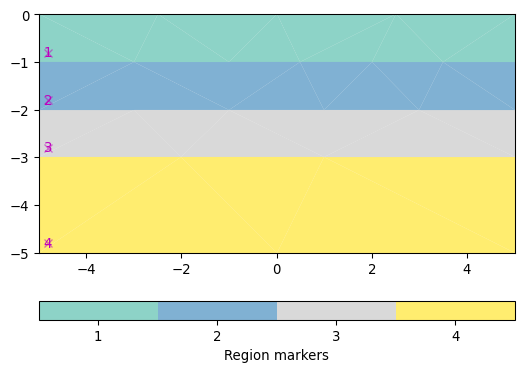### merge¶

pygimli.meshtools.merge(plcs, tol=0.001)

Merge multiply polygons.

Merge multiply polygons into a single polygon. Common nodes and common edges will be checked and removed. When a node touches an edge, the edge will be splited.

3D only OOC with polytools

Parameters
• plcs ([GIMLI::Mesh]) – List of PLC that want to be merged into one new PLC

• tol (double) – Tolerance to check for duplicated nodes. [1e-3]

Returns

plc – The resulting polygon is a GIMLI::Mesh.

Return type

GIMLI::Mesh

>>> import pygimli as pg
>>> import pygimli.meshtools as mt
>>> from pygimli.viewer.mpl import drawMesh
>>> world = mt.createWorld(start=[-10, 0], end=[10, -10], marker=1)
>>> c1 = mt.createCircle([-1, -4], radius=1.5, area=0.1,
...                       marker=2, nSegments=5)
>>> c2 = mt.createCircle([-6, -5], radius=[1.5, 3.5], isHole=1)
>>> r1 = mt.createRectangle(pos=[3, -5], size=[2, 2], marker=3)
>>> r2 = mt.createRectangle(start=[4, -4], end=[6, -6],
...                          marker=4, area=0.1)
>>> plc = mt.mergePLC([world, c1, c2, r1, r2])
>>> fig, ax = pg.plt.subplots()
>>> drawMesh(ax, plc)
>>> drawMesh(ax, mt.createMesh(plc))
>>> pg.wait()


(png, pdf)### merge2Meshes¶

pygimli.meshtools.merge2Meshes(m1, m2)[source]

Merge two meshes into one new mesh and return the combined mesh.

Merge two meshes into a new mesh and return the combined mesh. Note that there is a duplicate check for all nodes which should reuse existing node but NO cells or boundaries.

Parameters
Returns

mesh – Resulting mesh.

Return type

GIMLI::Mesh

### mergeMeshes¶

pygimli.meshtools.mergeMeshes(meshList, verbose=False)[source]

Merge several meshes into one new mesh and return the new mesh.

Merge several meshes into one new mesh and return the new mesh.

Parameters
• meshList ([GIMLI::Mesh, …] | [str, …]) – List of at least two meshes (or filenames to meshes) to be merged.

• verbose (bool) – Give some output

Examples using pygimli.meshtools.mergeMeshes### mergePLC¶

pygimli.meshtools.mergePLC(plcs, tol=0.001)[source]

Merge multiply polygons.

Merge multiply polygons into a single polygon. Common nodes and common edges will be checked and removed. When a node touches an edge, the edge will be splited.

3D only OOC with polytools

Parameters
• plcs ([GIMLI::Mesh]) – List of PLC that want to be merged into one new PLC

• tol (double) – Tolerance to check for duplicated nodes. [1e-3]

Returns

plc – The resulting polygon is a GIMLI::Mesh.

Return type

GIMLI::Mesh

>>> import pygimli as pg
>>> import pygimli.meshtools as mt
>>> from pygimli.viewer.mpl import drawMesh
>>> world = mt.createWorld(start=[-10, 0], end=[10, -10], marker=1)
>>> c1 = mt.createCircle([-1, -4], radius=1.5, area=0.1,
...                       marker=2, nSegments=5)
>>> c2 = mt.createCircle([-6, -5], radius=[1.5, 3.5], isHole=1)
>>> r1 = mt.createRectangle(pos=[3, -5], size=[2, 2], marker=3)
>>> r2 = mt.createRectangle(start=[4, -4], end=[6, -6],
...                          marker=4, area=0.1)
>>> plc = mt.mergePLC([world, c1, c2, r1, r2])
>>> fig, ax = pg.plt.subplots()
>>> drawMesh(ax, plc)
>>> drawMesh(ax, mt.createMesh(plc))
>>> pg.wait()


(png, pdf)Examples using pygimli.meshtools.mergePLC### mergePLC3D¶

pygimli.meshtools.mergePLC3D(plcs, tol=0.001)[source]

Merge a list of 3D PLC into one

Experimental replacement for polyMerge. Don’t expect too much.

Works if:
• all plcs are free and does not have any contact to each other

• contact of two facets if the second facet is completely within the first facet

### nodeDataToBoundaryData¶

pygimli.meshtools.nodeDataToBoundaryData(mesh, data)[source]

Assuming [NodeCount, dim] data DOCUMENT_ME

### nodeDataToCellData¶

pygimli.meshtools.nodeDataToCellData(mesh, data)[source]

Convert node data to cell data.

Convert node data to cell data via interpolation to cell centers.

Parameters
• mesh (GIMLI::Mesh) – 2D or 3D GIMLi mesh

• data (iterable [float]) – Data of len mesh.nodeCount(). TODO complex, R3Vector, ndarray

Examples

Examples using pygimli.meshtools.nodeDataToCellData### quality¶

pygimli.meshtools.quality(mesh, measure='eta')[source]

Return the quality of a given triangular mesh.

Parameters
• mesh (mesh object) – Mesh for which the quality is calculated.

• measure (quality measure, str) – Can be either “eta”, “nsr”, or “minimumAngle”.

>>> # no need to import matplotlib
>>> import pygimli as pg
>>> from pygimli.meshtools import polytools as plc
>>> from pygimli.meshtools import quality
>>> # Create Mesh
>>> world = plc.createWorld(start=[-10, 0], end=[10, -10],
...                         marker=1, worldMarker=False)
>>> c1 = plc.createCircle(pos=[0.0, -5.0], radius=3.0, area=.3)
>>> mesh = pg.meshtools.createMesh([world, c1], quality=21.3)
>>> # Compute and show quality
>>> q = quality(mesh, measure="nsr")
>>> ax, _ = pg.show(mesh, q, cMap="RdYlGn", showMesh=True, cMin=0.5,
...                 cMax=1.0, label="Normalized shape ratio")


(png, pdf)See also

eta(), nsr(), minimumAngle()

Examples using pygimli.meshtools.quality### readFenicsHDF5Mesh¶

pygimli.meshtools.readFenicsHDF5Mesh(fileName, group='mesh', verbose=True)[source]

Reads FEniCS mesh from file format .h5 and returns a GIMLI::Mesh.

### readGmsh¶

pygimli.meshtools.readGmsh(fName, verbose=False, precision=None)[source]

Read Gmsh ASCII file and return instance of GIMLI::Mesh class.

Parameters
• fName (string) – Filename of the file to read (\*.msh). The file must conform to the MSH ASCII file version 2 format

• verbose (boolean, optional) – Be verbose during import. Default: False

• precision (None|int, optional) – If not None, then round off node coordinates to the provided number of digits using numpy.round. This is useful in case that nodes are accessed using their coordinates, in which case numerical discrepancies can occur.

Notes

Physical groups specified in Gmsh are interpreted as follows:

• Points with the physical number 99 are interpreted as sensors. Note that physical point groups are ordered with respect to the node tag. For example, “Physical Point (99) = {50, 34};” and “Physical Point (99) = {34, 50};” will yield the same mesh. This must be taken into account when defining measurement configurations using electrodes defined in GMSH using marker 99.

• ERT only: Points with markers 999 and 1000 are used to mark calibration and reference nodes.

• Physical Lines and Surfaces define boundaries in 2D and 3D, respectively.
• Physical Number 1: Homogeneous Neumann condition

• Physical Number 2: Mixed boundary condition

• Physical Number 3: Homogeneous Dirichlet condition

• Physical Number 4: Dirichlet condition

• Physical Surfaces and Volumes define regions in 2D and 3D, respectively.
• Physical Number 1: No inversion region

• Physical Number >= 2: Inversion region

Examples

>>> import tempfile, os
>>> from pygimli.meshtools import readGmsh
>>> gmsh = '''
... $MeshFormat ... 2.2 0 8 ...$EndMeshFormat
... $Nodes ... 3 ... 1 0 0 0 ... 2 0 1 0 ... 3 1 1 0 ...$EndNodes
... $Elements ... 7 ... 1 15 2 0 1 1 ... 2 15 2 0 2 2 ... 3 15 2 0 3 3 ... 4 1 2 0 1 2 3 ... 5 1 2 0 2 3 1 ... 6 1 2 0 3 1 2 ... 7 2 2 0 5 1 2 3 ...$EndElements
... '''
>>> fName = tempfile.mktemp()
>>> with open(fName, "w") as f:
...     f.writelines(gmsh)
>>> mesh = readGmsh(fName)
>>> print(mesh)
Mesh: Nodes: 3 Cells: 1 Boundaries: 3
>>> os.remove(fName)


Examples using pygimli.meshtools.readGmsh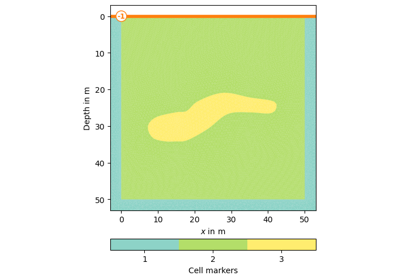### readHDF5Mesh¶

pygimli.meshtools.readHDF5Mesh(fileName, group='mesh', indices='cell_indices', pos='coordinates', cells='topology', marker='values', marker_default=0, dimension=3, verbose=True, useFenicsIndices=False)[source]

Function for loading a mesh from HDF5 file format.

Returns an instance of GIMLI::Mesh class. Default values for keywords are suited for FEniCS syntax .h5 meshes.

Requirements: h5py module

Parameters
• fileName (string) – Name of the mesh to be transformed into pyGIMLi format.

• group (string ['domains']) – hdf group that contains the mesh information (see other keyword arguments). Default is ‘domains’ for FEniCS compatibility.

• indices (string ['cell_indices']) – Key for the part of the hdf file containing the indices of the cells.

• pos (string ['coordinates']) – Key for the part of the hdf file containing the nodepositions.

• cells (string ['topology']) – Key for the part of the hdf file containing the array which defies the cells. Usually of shape (cellCount, 3) for 2D meshes or (cellCount, 4) for 3D tetrahedra meshes. For each cell the indices of the corresponding node indices is given.

• marker (string ['values']) – If marker is part of the hdf data container, the corresponding array is used as identifier for the cell markers. If not found, the cell markers will be set to marker_default.

• marker_default (int or array ) – Default marker if no markers are found in the hdf file. If array, size has to match the cellCount of the mesh.

• dimension (int ) – Dimension of the input/output mesh, no own check for dimensions yet. Fixed on 3 for now.

Returns

GIMLI::Mesh

Return type

mesh

### readHydrus2dMesh¶

pygimli.meshtools.readHydrus2dMesh(fileName='MESHTRIA.TXT')[source]

Import mesh from Hydrus2D.

Parameters

fName (str, optional) – Filename of Hydrus output file.

See also

readHydrus3dMesh()

Similar routine for three-dimensional meshes.

References

### readHydrus3dMesh¶

pygimli.meshtools.readHydrus3dMesh(fileName='MESHTRIA.TXT')[source]

Import mesh from Hydrus3D.

Parameters

fName (str, optional) – Filename of Hydrus output file.

See also

readHydrus2dMesh()

Similar routine for two-dimensional meshes.

References

### readMeshIO¶

pygimli.meshtools.readMeshIO(fileName, verbose=False)[source]

Generic mesh read using meshio. (https://github.com/nschloe/meshio)

### readPLC¶

pygimli.meshtools.readPLC(filename, comment='#')[source]

Read in a piece-wise linear complex object, i.e. pyGIMLi geometry, from .poly file. The latter could be created with mt.exportPLC.

Read 2D Triangle or 3D Tetgen PLC files.

Parameters
• filename (string) – Filename *.poly

• comment (string ('#')) – String containing all characters that define a comment line. Identified lines will be ignored during import.

Returns

GIMLI::Mesh

Return type

poly

### readSTL¶

pygimli.meshtools.readSTL(fileName, binary=False)[source]

Read STL surface mesh and returns a GIMLI::Mesh.

Read STL surface mesh and returns a GIMLI::Mesh of triangle boundary faces. Multiple solids are supported with increasing boundary marker.

TODO: ASCII=False, read binary STL

Parameters
• fileName (str) – name of the .stl file containing the STL surface mesh

• binary (bool [False]) – STL Binary format

### readTetgen¶

pygimli.meshtools.readTetgen(fName, comment='#', verbose=False, defaultCellMarker=0, loadFaces=True, quadratic=False)[source]

Read and convert a mesh from the basic Tetgen output.

Read Tetgen [Si04] ASCII files and return instance of GIMLI::Mesh class. See: http://tetgen.org/

Parameters
• fName (str) – Base name of the tetgen output, without ending. All additional files (.node, .ele and .face) need to have the same basename.

• comment (str ('#')) – String consisting of all symbols indicating a comment in the input files. Standard for tetgen files is the ‘#’.

• verbose (boolean (True)) – Enables console output during the import process.

• defaultCellMarker (int (0)) – Tetgen files can contain cell markers, but do not have to. If no markers are found, the given integer is used.

• loadFaces – Optional decision whether the faces of Tetgen output (.face) are loaded or not. Note that without the -f in during the tetgen call, the faces in the .face file will only contain the faces of the original input poly file and not all faces. If only a part of the faces are imported, a createNeighborInfos call of the mesh will fail.

• quadratic (boolean (False)) – Returns a P2 (quadratic) refined mesh when True (to be removed, as soon as direct import of quadratic meshes is possible).

Returns

mesh

Return type

GIMLI::Mesh

### readTriangle¶

pygimli.meshtools.readTriangle(fName, verbose=False)[source]

Read Triangle [She96] mesh.

Read Triangle [She96] ASCII mesh files and return an instance of GIMLI::Mesh class. See: ://www.cs.cmu.edu/~quake/triangle.html

Parameters
• fName (string) – Filename of the file to read (\*.n, \*.e)

• verbose (boolean, optional) – Be verbose during import.

### refineHex2Tet¶

pygimli.meshtools.refineHex2Tet(mesh, style=1)[source]

Refine mesh of hexahedra into a mesh of tetrahedra.

Parameters
• mesh (GIMLI::Mesh) – Mesh containing hexrahedron cells, e.g., from a grid.

• style (int ) –

• 1 bisect each hexahedron int 6 tetrahedrons (less numerical quality but no problems due to diagonal face split)

• 2 bisect each hexahedron int 5 tetrahedrons (will lead to inconsistent meshes. Neighboring cell will have different face split diagonal. Might be fixable by rotating the split order depending on coordinates for every 2nd split .. if someone really need this.)

Returns

ret – Mesh containing tetrahedrons cells.

Return type

GIMLI::Mesh

Examples

>>> import pygimli as pg
>>> import pygimli.meshtools as mt
>>> hex = pg.createGrid(2, 2, 2)
>>> print(hex)
Mesh: Nodes: 8 Cells: 1 Boundaries: 6
>>> tet = mt.refineHex2Tet(hex, style=1)
>>> print(tet)
Mesh: Nodes: 8 Cells: 6 Boundaries: 12
>>> tet = mt.refineHex2Tet(hex, style=2)
>>> print(tet)
Mesh: Nodes: 8 Cells: 5 Boundaries: 12


### refineQuad2Tri¶

pygimli.meshtools.refineQuad2Tri(mesh, style=1)[source]

Refine mesh of quadrangles into a mesh of triangle cells.

TODO mixed meshes

Parameters
• mesh (GIMLI::Mesh) – Mesh containing quadrangle cells.

• style (int ) –

• 1 bisect each quadrangle into 2 triangles

• 2 cross-sect each quadrangle into 4 triangles

Returns

ret – Mesh containing triangle cells.

Return type

GIMLI::Mesh

>>> import pygimli as pg
>>> import pygimli.meshtools as mt
>>> quads = pg.createGrid(range(10), range(10))
>>> ax, _ = pg.show(quads)
>>> ax, _ = pg.show(mt.refineQuad2Tri(quads, style=1))
>>> ax, _ = pg.show(mt.refineQuad2Tri(quads, style=2))
>>> pg.wait()(png, pdf)(png, pdf)(png, pdf)

### syscallTetgen¶

pygimli.meshtools.syscallTetgen(filename, quality=1.2, area=0, preserveBoundary=False, verbose=False, tetgen='tetgen')[source]

Create a mesh with Tetgen from file.

Create a Tetgen [Si04] mesh from a PLC.

Forwards to system call tetgen, which must be known to your system.

Parameters
• filename (str) –

• quality (float [1.2]) – Refines mesh (to improve mesh quality). [1.1 … ]

• area (float [0.0]) – Maximum cell size (m³)

• preserveBoundary (bool [False]) – Preserve PLC boundary mesh

• verbose (bool [False]) – be verbose

• tetgen (str | path ['tetgen']) – Binary for tetgen. Given as complete path or simple the binary name if its known in the system path.

Returns

mesh

Return type

GIMLI::Mesh

### tapeMeasureToCoordinates¶

pygimli.meshtools.tapeMeasureToCoordinates(tape, pos)[source]

Interpolate 2D tape measured topography to 2D Cartesian coordinates.

Tape and pos value are expected to be sorted along distance to the origin.

DEPRECATED will be removed, use pygimli.meshtools.interpolateAlongCurve` instead

TODO optional smooth curve with harmfit TODO parametric TODO parametric + Topo: 3d

Parameters
• tape ([[x,z]] | [RVector3] | R3Vector) – List of tape measured topography points with measured distance (x) from origin and height (z)

• pos (iterable) – Array of query positions along the tape measured profile t[0 ..

Returns

res – Same as pos but with interpolated height values. The Distance between pos points and res (along curve) points remains.

Return type

ndarray(N, 2)